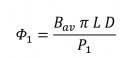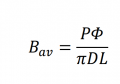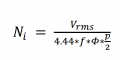# magnetic flux per pole

#### Eric M

Joined Sep 19, 2023
1
Hello. I design a PMSM surface mounted motor with 8 poles and 48 slots, single layer winding. I want to calculate how many turns per slot .
I know also that D (130mm) and L (100mm) .

the first equations i need is :When i find out Magnetic Flux per pole and B average, then i can calculate the number of turns per coil by this equation:The question is :
What is Φ (Magnetic flux per pole), and what is the difference between Bav?

How can i calculate them (Φ,Bv) with the software called FEMM ?